Cheng, L., Dai, J., Yang, Z., Qian, W., Wang, W., Chang, L., Li, X., and Wang, Z. (2020). "Theoretical and experimental research on moisture content and wood property indexes based on nondestructive testing," BioRes. 15(1), 1600-1616.

#### Abstract

A set of reformulated theoretical formulas was developed to measure the relationships between moisture content (MC) and stress wave propagation velocity, dynamic modulus of elasticity ( “E” _”d” ), and modulus of stress-resistograph of the wood. The theory of wood science, elastic mechanics, wave science, and stress wave propagation were used as the theoretical basis. Using larch as the material, both stress wave and micro-drilling resistance technologies were used to study the timber property changes under different moisture contents. The results showed that when the MC of wood did not reach the fiber saturation point (FSP), the wood property decreased sharply with increased MC. However, the study also found that when the MC of wood was higher than the FSP, the wood property decreased with increased MC. In addition, the experimental results showed that the variation trend calculated by the new set of theoretical formulas was consistent with the numerical variation trend measured by the experiment, and the coupling effect of stress wave and micro-drilling resistance was high. This set of theoretical formulas can provide a reference for the research on nondestructive testing and performance evaluation of ancient building timber.

Theoretical and Experimental Research on Moisture Content and Wood Property Indexes Based on Nondestructive Testing

Liting Cheng,a,f Jian Dai,b,f,* Zhiguo Yang,c Wei Qian,b,f,* Wei Wang,b Lihong Chang,d Xin Li,e and Ziyi Wang a

A set of reformulated theoretical formulas was developed to measure the relationships between moisture content (MC) and stress wave propagation velocity, dynamic modulus of elasticity (), and modulus of stress-resistograph of the wood. The theory of wood science, elastic mechanics, wave science, and stress wave propagation were used as the theoretical basis. Using larch as the material, both stress wave and micro-drilling resistance technologies were used to study the timber property changes under different moisture contents. The results showed that when the MC of wood did not reach the fiber saturation point (FSP), the wood property decreased sharply with increased MC. However, the study also found that when the MC of wood was higher than the FSP, the wood property decreased with increased MC. In addition, the experimental results showed that the variation trend calculated by the new set of theoretical formulas was consistent with the numerical variation trend measured by the experiment, and the coupling effect of stress wave and micro-drilling resistance was high. This set of theoretical formulas can provide a reference for the research on nondestructive testing and performance evaluation of ancient building timber.

Keywords: Nondestructive testing; Larch; Moisture content; Stress wave; Micro-drilling resistance; Modulus of elasticity; Modulus of stress-resistograph

Contact information: a: College of Architecture and Civil Engineering, Beijing University of Technology, Beijing 100124, China; b: College of Architecture and Urban Planning, Beijing University of Technology, Beijing 100124, China; c: College of Petroleum Engineering, China University of Petroleum (Beijing), Beijing 102249, China; d: College of Urban and Rural Development, Beijing University of Agriculture, Beijing 102206, China; e: College of Architecture and Art, North China University of Technology, Beijing 100144; f: Beijing Engineering Technology Research Center for Historic Building Protection, Beijing University of Technology, Beijing 100124, China; *Corresponding author:chenglting@126.com

INTRODUCTION

Wood is an important building material. It plays an important supporting role in the whole architecture system of ancient wooden buildings (Ni et al. 2019). Because wood is an anisotropic material, there are many factors that affect wood properties, including density, ring, texture, sapwood difference, moisture content (MC), and other defects (Liu et al. 2014). Wood is also susceptible to moisture (Slavik et al. 2019). The MC affects the strength, rigidity, and volume stability of the wood within a certain range (Gao et al. 2014).

Accurately testing the properties of timber plays a vital role in the safety monitoring and maintenance of ancient building wood members. The commonly used nondestructive testing (NDT) technologies for wood properties include the longitudinal stress wave technology and the micro-drilling resistance testing technology (Zhang et al. 2011). The longitudinal stress wave technology estimates the and predicts mechanical strength and properties of wood (Guan et al. 2013b). The micro-drilling resistance detection technology detects material changes in wood (Li et al. 2016). The combined use of the two technologies can better detect wood properties (Zhu et al. 2011).

However, wood MC can influence the accuracy of the two above technologies (Liao et al. 2012). The stress wave propagation velocity is a vital detection index in the stress wave technology. The MC has a significant effect on stress wave propagation velocity (Zhu 2012), and the acoustic propagation velocity is negatively correlated with MC within a certain range (Si and Lu 2007; Liu and Gao 2014; Montero et al. 2015; Peng et al. 2016). The estimated from the stress wave propagation velocity and density also decreases to various degrees with the increase of MC (Moreno-Chan et al. 2011; Chang 2017). The degree of decrease is related to the influence of MC on the direction (Chen et al. 2012; Wei et al. 2019), path of stress wave propagation (Guan et al. 2013a), and the temperature (Wang et al. 2008, 2009; Gao et al. 2009, 2010) in wood. The influences of MC on stress waves propagation velocity and on the are considerably different (Nocetti et al. 2015; Llana et al. 2018). The difference between and the static modulus of elasticity (MOE) tends to be constant when MC is above FSP (Wang 2008).

The micro-drilling resistance values (including the rotational resistance value of the drilling needle (fdrill) and the resistance value of the feeding needle (ffeed) are two important detection indexes in the micro-drilling resistance detection technology. The MC affects the size of the resistance. Within a certain range, the resistance may first increase and then decrease with the increase of MC (Sun 2012). Much research has been conducted to evaluate the relationship between MC and wood property detection indexes. The examples include using a ultrasound tester (Gonçalves and Leme 2008; Gonçalves et al. 2018), a stress wave tester (Si and Lu 2007), micro-drilling resistance instrument (Sun 2012), or the combined use of the last two in the studies on different tree species (Liao et al. 2012), different degrees of decay (Li 2015), and different profiles (Brashaw et al. 2004; Carter et al. 2005).

This study investigated the in-depth relationship between MC and wood mass testing indicators. This study was developed based on a) the research results of elasticity theory (Yu et al. 2014), wave theory (Yang and Wang 2005), and stress wave longitudinal propagation theory (Liu 2014; Dackermann et al. 2016), combining the theory of wood and wave propagation; b) the testing technology of stress wave and micro-drilling resistance; and c) the key factors that affect wood properties. In this study, the representative larch specimens were selected as the research material, and the experimental data and the calculated value of the theoretical formula were verified. The results show that the two values and trends are consistent. Thus, this study also developed a set of reformulated theoretical formulas for the relationship between MC and stress wave propagation velocity, , and modulus of stress-resistograph (including the modulus of stress-resistograph of the drilling needle (Fdrill) and the modulus of stress-resistograph of the feeding needle (Ffeed). This set of reformulated theoretical formulas provides a reference for timber property detection and preventive protection research of ancient wood architectural members to conduct the nondestructive testing, monitoring, surveying, and repairing of ancient timber structures.

Theory of MC and wood property indexes based on NDT

Dry wood is mainly composed of wood fiber structures. When the MC increases, water molecules preferentially entered the wood fiber structure in the state of combined water. This is the hydrophilic and self-imbibition effect of the wood fiber. When the fiber structure is full of water molecules, the wood FSP is reached. Then, the water molecules continuously fill in the structural gaps between the wood fibers and exist in the state of free water (Fig. 1) (The Kingdom of Wood 2018).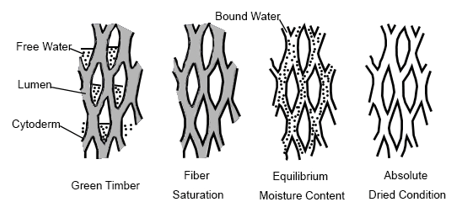Fig. 1. The state of existence of water in the wood

When the medium in the wood is a continuum, the parameters, such as the density and , of the medium are continuous. The FSP is approximately 30% accordingly. Considering the swelling of the wood after it has absorbed water, the volume of the wood after water absorption is shown in Eq. 1,

V = (1 + KWV(1)

where is the volume after wetting (cm3), V0 is the volume of wood when absolutely dry (cm3), K is the wet expansion coefficient (%),K = S / WS is the full wet expansion rate of wood (%), S = (( V0) / V0) × 100, and W is the difference value between the test and the MC of the wood at the beginning of the test (when the MC is lower than 30%, W is the difference value between the test and the MC of the wood at the beginning of the test; when the MC is greater than 30%, it is 30 (%).

The calculation formula based on density, d = m / v, the density of the wood is shown in Eq. 2,

ρc = (mo (1 + ω)) / ((1 + KWV0) (2)

where ρc is the density of wood (g/cm3), mo is the mass of wood substance (g), ω is the MC (absolute MC) (%), ω = ((m – mo) / m0) × 100 = ((m / mo) – 1) × 100, ma is the mass of water in wood (g), and m is the total mass of wood (g), m = mo + ma (the mass of the air is small, and is therefore ignored).

The stress waves used in the field of NDT of wood are mostly shock stress waves generated by hammering (Xu 2011). The propagation of stress waves in wood is influenced by properties, direction, and microstructure of wood. It is also closely related to the physical and mechanical properties of wood. There are three common ways the stress waves propagate inside wood: longitudinal propagation, radial propagation, and lateral propagation. Using stress wave technology, the stress waves enter from two-end faces. This belongs to the uniaxial forward propagation and is a one-dimensional stress wave state. Studies (Bertholf 1965) have tested the validity of the hypothesis. Combined with the calculation formula of the longitudinal wave velocity in the propagation theory of stress waves in anisotropic infinite elastic body (Timoshenko and Doodier 1970; Carino and Sansalone 1991), the wave velocity of the stress wave in the wood is shown in Eq. 3,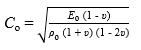(3)

where Co is the stress wave propagation velocity in wood (m/s), Eis the modulus of elasticity of wood (MPa), and υ is the Poisson’s ratio.

According to the hypothetical model proposed by Gao (2012), it is assumed that wood is the macroscopic composition of the mixture. The moisture in the wood is all free when the temperature is above 0 °C. The macroscopic view of the wood is composed of wood substance and water. When the MC is ω, the acoustic wave velocity in the wood is shown in Eq. 4,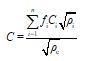(4)

where is the sound wave propagation velocity in wood (m/s),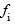is the content of each mixed component of wood (%),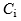is the speed of sound waves in each mixed component (m/s),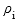is the density of each mixed component (kg/m3), and n is 2.

According to Eq. 4, the stress wave propagation velocity in wood is shown in Eq. 5,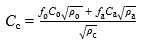(5)

where Cis the stress wave propagation velocity in wood (m/s), Ca is the stress wave propagation velocity in water (m/s), fo is the content of wood substance (%),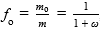, fa is the content of water in wood (%),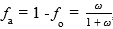, ρa is the density of water (g/cm3), and ρo is the density of wood substance (g/cm3).

According to the conversion relationship between fofa, and ω, the stress wave propagation velocity is shown in Eq. 6: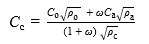(6)

The of wood after it absorbs water is shown in Eq. 7,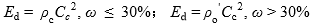(7)

where Ed is the dynamic modulus of elasticity of wood (MPa),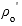is the density of the cell wall and wood substance (g/cm3),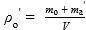, and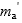is the mass of sucking water (g), when the wood reaches the FSP,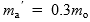.

According to the modulus of stress-resistograph defined by Zhu Lei et al. (2011), combined with Eq. 6 and the rotational resistance value of the drilling needle of the micro-drilling resistance instrument, the modulus of stress-resistograph of the drilling needle (Fdrill) is shown in Eq. 8,

Fdrill = fdrill × Cc2 (8)

where Fdrill is the modulus of stress-resistograph of the drilling needle (resi·km2/m2), and fdrill is the rotational resistance value of the drilling needle (resi).

Combining the Eq. 6 and the resistance value of the feeding needle of the micro-drilling resistance instrument, the modulus of stress-resistograph of the feeding needle (Ffeed) is shown in Eq. 9,

Ffeed = ffeed × Cc(9)

where Ffeed is the modulus of stress-resistograph of the feeding needle (resi·km2/m2), and ffeed is the resistance value of the feeding needle (resi).

EXPERIMENTAL

Materials

Larch wood (Larix gmelinii) was selected and used as the experimental material and was purchased from Eastern Royal Tombs of the Qing Dynasty Wood Factory (Tangshan City, Hebei Province, China). The wood is about 300 to 400 years old. According to the standard on the size and method of acquisition requirements for test specimens GB 1929 (2009), the sample was sawn into specimens with a size of 2 cm × 2 cm × 36 cm. Small test specimens of 2 cm × 2 cm × 2 cm were cut from each large test specimen. The specimens of 2 cm × 2 cm × 30 cm were tested for stress wave and micro-drill resistance at different moisture contents, (Figs. 2 and 3).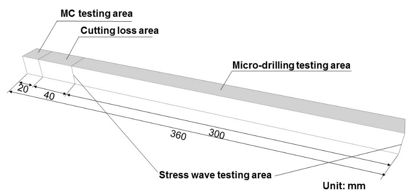Fig. 2. Experimental specimen partition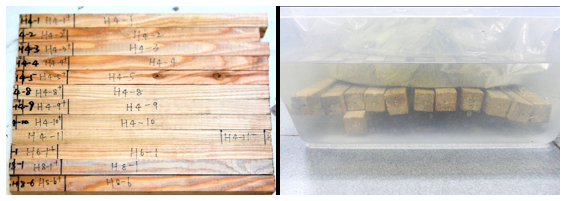Fig. 3. Experimental test specimens

The method of sawing the sample was perpendicular to the direction of rings (Fig. 4). There were 12 specimens. They were from the same log. As one part of the wood was exposed to light and the other part was not during growing, the 12 specimens were divided into two parts, light part and shade part.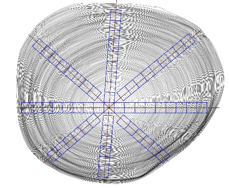Fig. 4. The method of sawing the sample

Methods

Experiment apparatus

The apparatuses used were a Lichen Technology blast dryer box 101-3BS, Lichen Technology electronic precision balance JA1003 (Shanghai Lichen Electronic Technology Co., Ltd., Shanghai, China), FAKOPP microsecond timber (FAKOPP Enterprise Bt., Ágfalva, Hungary), and an IML-RESI PD500 micro-drill resistance instrument (IML Co., Ltd., Wiesloch, Germany).

Methods and steps

The mass of each small specimen block was measured after drying in the dryer box (Fig. 5). The MC of the test specimens was determined according to GB 1931 (1991) (Fig. 6).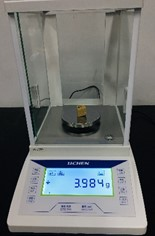Fig. 5. Small specimen weighing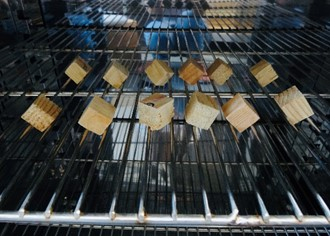Fig. 6. Small test specimens drying

At indoor temperature, the test specimens were immersed at intervals of 2 min (0.03 h), 3 min (0.05 h), 5 min (0.08 h), 10 min (0.17 h), 15 min (0.25 h), 30 min (0.5 h), until 96,000 min (1600 h). The mass of the test specimens was measured by an electronic precision balance after each soaking (Fig. 7). And the mass of each test specimen was carried out by mass difference between the mass of the full specimen and the mass of 2cm cubic specimen dried in the oven.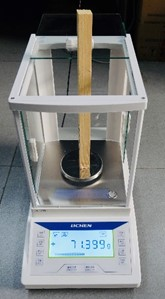Fig. 7. Specimen weighing

The stress wave was used to measure the propagation time of stress waves in the wood. In this study, the two probes of the instrument were inserted into the two ends of the test specimen and measured along the longitudinal direction of the test specimen. The angle between the two probes and the longitudinal axis of the test specimen was no less than 45°. The distance between the two points was measured (Fig. 8). The propagation time readings of all the taps were measured except the first tap, in which the data was found invalid. The results were then calculated by measuring the average value of three continuous tapping times from the second tap. The propagation velocity of the stress wave in the test specimen was calculated using Eq. 10,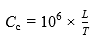(10)

where is the distance between the two sensors of the stress wave tester (m), and is the time recorded between the two sensors of the stress wave tester (μs). The dynamic elastic modulus of wood was calculated using Eq. 11: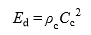(11)

The resistance value of the wood interior material was measured using an IML-RESI PD500 micro-drill resistance instrument. One probe was drilled into the surface of the finished test specimen at a constant speed and perpendicular to the annual ring direction. This is shown in Fig. 9. The data were then entered into the computer to calculate the resistance value. The wave resistance modulus was also calculated, combining the micro-drill resistance values and the stress wave propagation speed (see Eq. 12 and Eq. 13):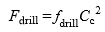(12)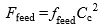(13)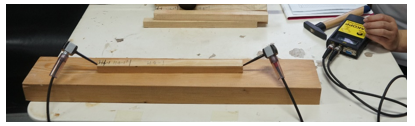Fig. 8. Determination of stress wave propagation time of specimen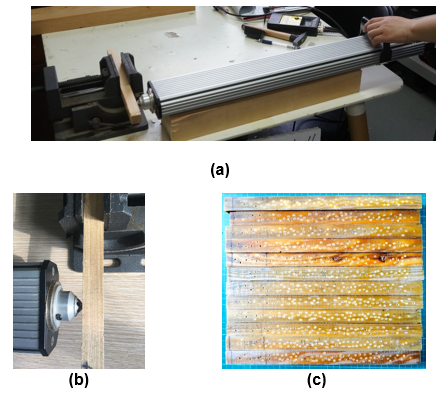Fig. 9. Determination of the resistance values of the micro-drilling of the test specimens

RESULTS AND DISCUSSION

The selected wood specimens were divided into the shade part and light part. The average data was collected by measuring the shade and light parts to represent the overall timber properties. The MC of wood specimens changed with time (Fig. 10). The trend of wood specimens’ density with MC is shown in Fig. 11.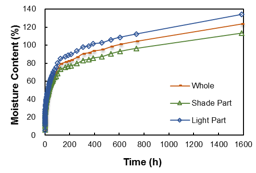Fig. 10. MC changes with time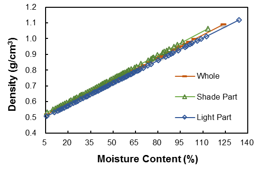Fig. 11. Density changes with MC